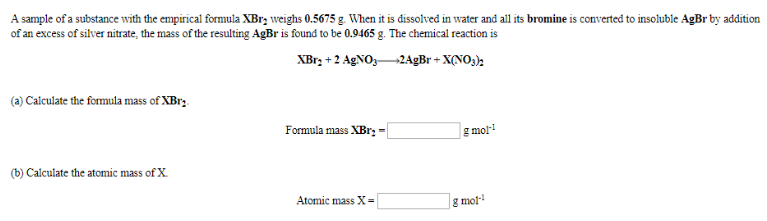# Problem: A sample of a substance with the empirical formula XBr2 weighs 0.5675 g. When it is dissolved in water and all its bromine is converted to insoluble AgBr by addition of an excess of silver nitrate, the mass of the resulting AgBr is found to be 0.9465 g. The chemical reaction is XBr2 + 2AgNO3 → 2AgBr + X(NO3)2 (a) Calculate the formula mass of XBr2.  (b) Calculate the atomic mass of X.

###### FREE Expert Solution
98% (340 ratings)###### Problem Details

A sample of a substance with the empirical formula XBr2 weighs 0.5675 g. When it is dissolved in water and all its bromine is converted to insoluble AgBr by addition of an excess of silver nitrate, the mass of the resulting AgBr is found to be 0.9465 g. The chemical reaction is

XBr2 + 2AgNO3 → 2AgBr + X(NO3)2

(a) Calculate the formula mass of XBr2.

(b) Calculate the atomic mass of X.